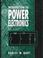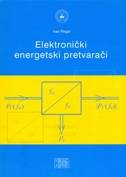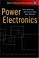### Power Electronics Practicum

#### Course Description

Getting acquainted with real solutions of power converters and their operating characteristics. Power electronic converters, interface between power source and load. Topologies and functions; basic power conversions. Power converter components; semiconductor and magnetic, power switches, drivers, DC and AC filters. Power converter modeling and simulation; control and power characteristics measurement. Protection and cooling. Power converter application examples on laboratory devices; line converters, DC/DC and AC/AC converters, inverters.

#### General Competencies

Knowing fundamental topologies and functions of power electronic converters. Ability to select appropriate converter according to required application. Ability of converter commissioning.

#### Learning Outcomes

1. explain operation principles of basic power electronic converters
2. apply the acquired knowledge for modeling and simulation of basic power converters.
3. apply the acquired knowledge to measure the characteristic waveforms of the basic power converters
4. modify the simulation model to obtain model behavior closer to the real circuit.
5. analyze the operation of basic power converters using simulation results and measurements.
6. analyze the complex system of a power converters on the basic components.

#### Forms of Teaching

Lectures

Lectures are organized through 2 teaching cycles. The first cycle consists of 7 weeks of classes and mid-term exam, a second cycle of 6 weeks of classes and final exam. Classes are conducted through a total of 15 weeks with a weekly load of 1 hour.

Exams

Examination consists of mid-term exam and final exam in which numerical problems are solved, and the writing of reports and seminars about laboratory exercises.

Laboratory Work

Laboratory exercises are organized through 2 cycles. The first cycle consists of 4 exercises, a second cycle of 2 exercises. Exercises are conducted through a total of 15 weeks with a weekly load of 2 hours.

Seminars

At the end of the second cycle of lectures, students are writting ​​seminars which demonstrate the ability to connect theoretical knowledge, modeling and simulation and analysis of measurement results.

Continuous Assessment Exam
Laboratory Exercises 0 % 30 % 0 % 30 %
Seminar/Project 0 % 30 % 0 % 30 %
Mid Term Exam: Written 10 % 10 % 0 %
Final Exam: Written 10 % 20 %
Final Exam: Oral 10 %
Exam: Written 10 % 20 %
Exam: Oral 20 %

#### Week by Week Schedule

1. Fundamentals of power electronics circuits simulation.
2. Fundamentals of power electronics circuits measurements.
3. Basic power electronics switches. Idealised characteristics of power switches.
4. Power converters topologies and circuits. Rectification and DC/DC conversion.
5. Power converters topologies and circuits. Inversion and AC/AC conversion.
6. Converter control and power characteristics, efficiency, power factor, displacement factor.
7. Midterm exam
8. Interaction of power converter, power supply and the load.
9. Power converter system for renewable energy sources as complex power electronic system. Analysis of basic components.
10. Power converter system for renewable energy sources as complex power electronic system. Digital control system analysis. Properties and abilities. Practical work with system.
11. Driver circuits for thyristors and transistors (bipolar, MOSFET and IGBT).
12. Power semiconductor's dissipated power calculation. Thermal management. Case study.
13. Power semiconductors protection, snubbers, fuses. Catalogue data interpretation and application.
14. Presentation of seminars. Analysis of complex power electronic systems.
15. Final exam

#### Study Programmes

Control Engineering and Automation (module)
Elective Courses (6. semester)
Electrical Power Engineering (module)
Elective Courses (6. semester)

#### LiteratureD. W. Hart (1997.), Introduction to Power Electronics, Prentice HallB. W. Williams (2006.), Principles and Elements of Power Electronics, B. W. WilliamsI. Flegar (2010.), Elektronički energetski pretvarači, KigenN. Mohan, T. Undeland, W. Robins (2004.), Power Electronics: Converters, Applications and Design, Wiley

#### General

ID 34347
Summer semester
4 ECTS
L1 English Level
L1 e-Learning
15 Lectures
30 Laboratory exercises